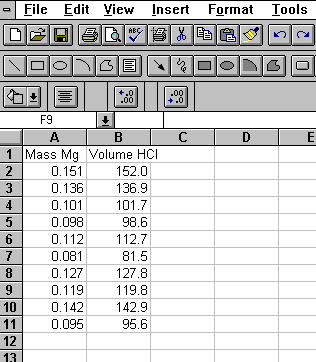## Introduction to Microsoft Excel

Microsoft Excel is a tool which has many uses, the most common of which are performing calculations and plotting graphs.

When you open Microsoft Excel you will see a grid. Each box in the grid is called a cell. Each cell has an "address" made up of a letter indicating the column the cell is in and a number indicating the row the cell is in. For example, the upper left cell is A1.

The first step in doing a spreadsheet calculation or making a graph is entering the data.

For example, if we did an experiment reacting several different samples of magnesium metal with hydrochloric acid, we might want to set up a spreadsheet containing the mass of magnesium and volume of hydrochloric acid used. We would create a spreadsheet that looks like figure 1.